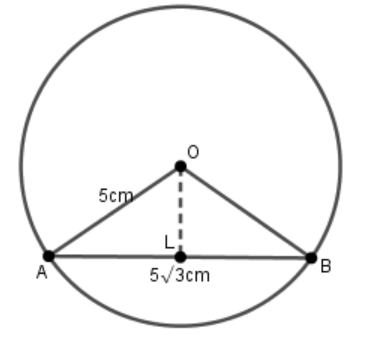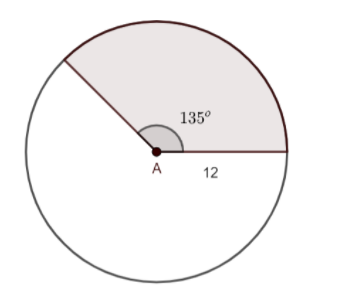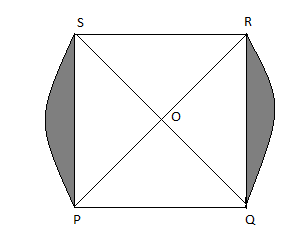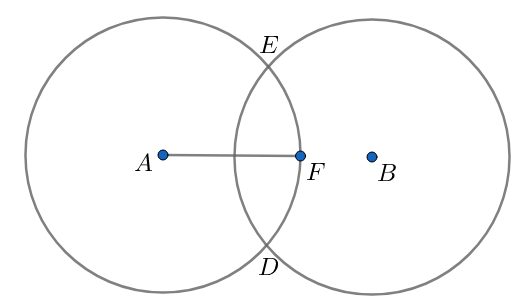Filters
Latest Questions
Mathematics
Areas of sector of a circle
Find the area of the sector of a circle of radius 4 cm and angle ${30^ \circ }$. Find the area of the corresponding major sector. $(\pi = 3.14)$

Mathematics
Areas of sector of a circle
AB and CD are arcs of the two concentric circles of radii 21cm and 7cm and centre ‘O’ if $\angle \text{AOB}={{30}^{\circ }}$. Find the area of the shaded region.Mathematics
Areas of sector of a circle
A rectangular piece is $20m$ long and $15m$ wide. From its four corners, quadrants of radii $3.5m$ have been cut. Find the area of the remaining part.

Mathematics
Areas of sector of a circle
In a circle with centre O and radius $5cm$, AB is a chord of length $5\sqrt 3 cm$. Find the area of sector AOB.A). $\dfrac{{25\pi }}{2}c{m^2}$
B). $\dfrac{{23\pi }}{3}c{m^2}$
C). $\dfrac{{25\pi }}{7}c{m^2}$
D). None of these

Mathematics
Areas of sector of a circle
What is the area of the sector?A. $170\,c{{m}^{2}}$
B. $100\,c{{m}^{2}}$
C. $110\,c{{m}^{2}}$
D. $130\,c{{m}^{2}}$

Mathematics
Areas of sector of a circle
Find the area of the sector of a circle of radius 5 cm, if the corresponding arc length is 3.5 cm.

Mathematics
Areas of sector of a circle
Write the formula to find the area of a sector and length of an arc for the following:
(a) Semicircle
(b) Quarter circle

Mathematics
Areas of sector of a circle
In figure, PQRS is a square lawn with side PQ = 42 metres. Two circular flowerbeds are there on the sides PS and QR with centre at O, the intersection of its diagonals. Find the total area of the two flower beds (shaded parts).Mathematics
Areas of sector of a circle
OPQ is the sector of a circle having a center at O and radius 15cm. If $m\angle POQ={{30}^{\circ }}$, find the area enclosed by arc PQ and chord PQ.
Mathematics
Areas of sector of a circle
How do you calculate the length of an arc and area of a sector?

Mathematics
Areas of sector of a circle
Two overlapping circles with equal radius form a shaded region as shown in the figure. Express the area of the region and the complete perimeter (combined arc length) in terms of r and the distance between centre D? Let $r=4$ , $D=6$ and calculate?Mathematics
Areas of sector of a circle
The minute hand of a clock is 8 cm long. Find the area swept by the minute hand between 8.30 a.m, and 9.05 a.m.
A.$177\dfrac{1}{3}c{{m}^{2}}$
B.$107\dfrac{1}{3}c{{m}^{2}}$
C.$217\dfrac{1}{3}c{{m}^{2}}$
D. None of these

Prev
1
2
3
4